# 条形码

## 正文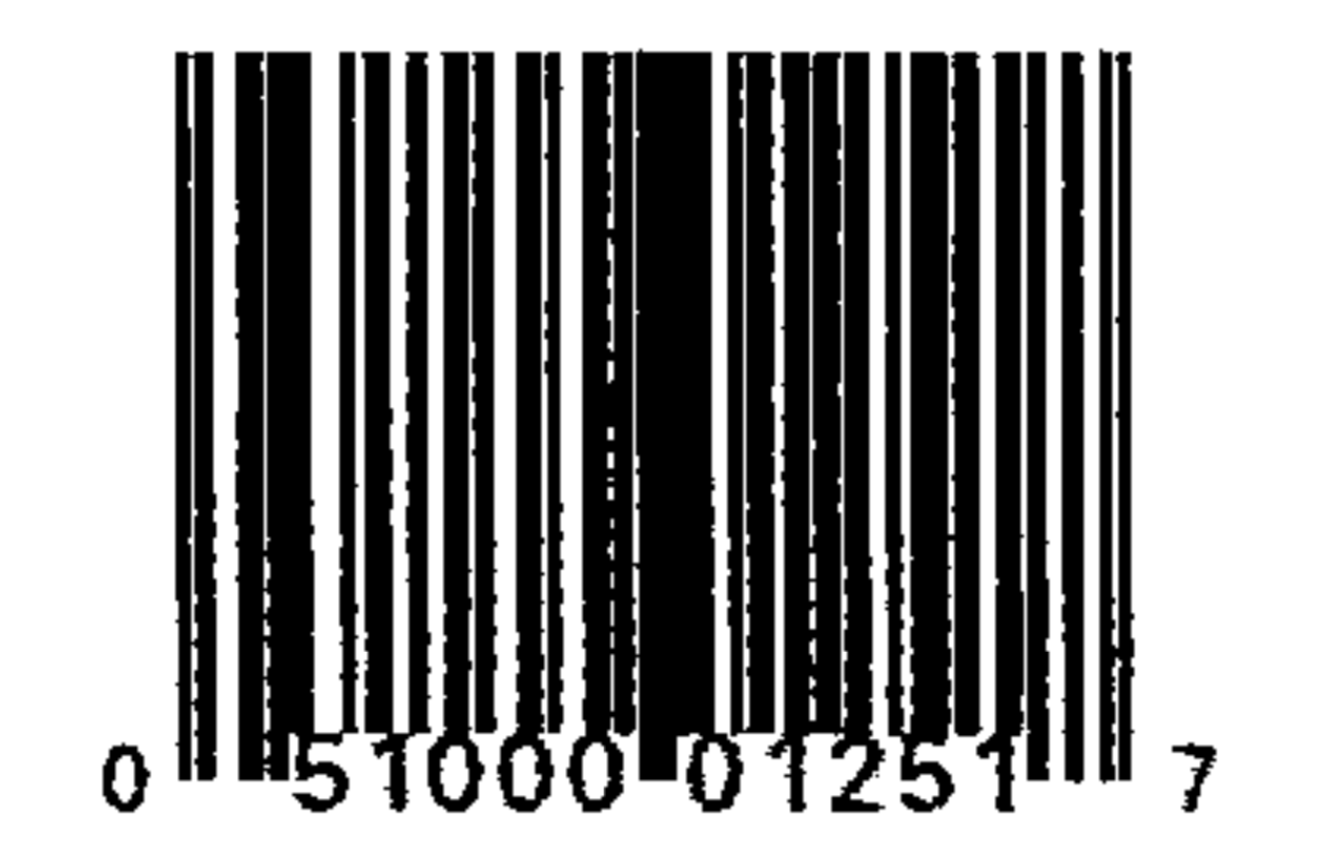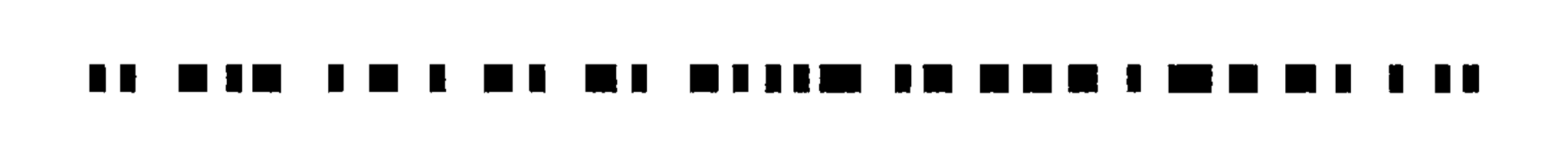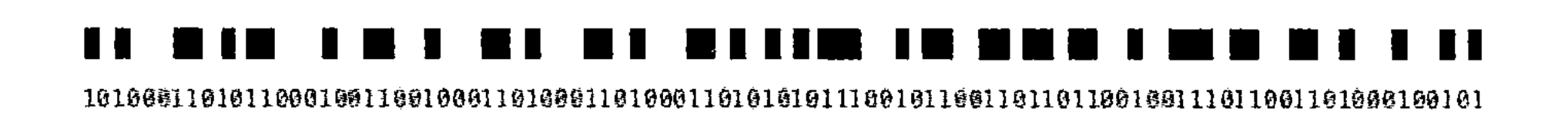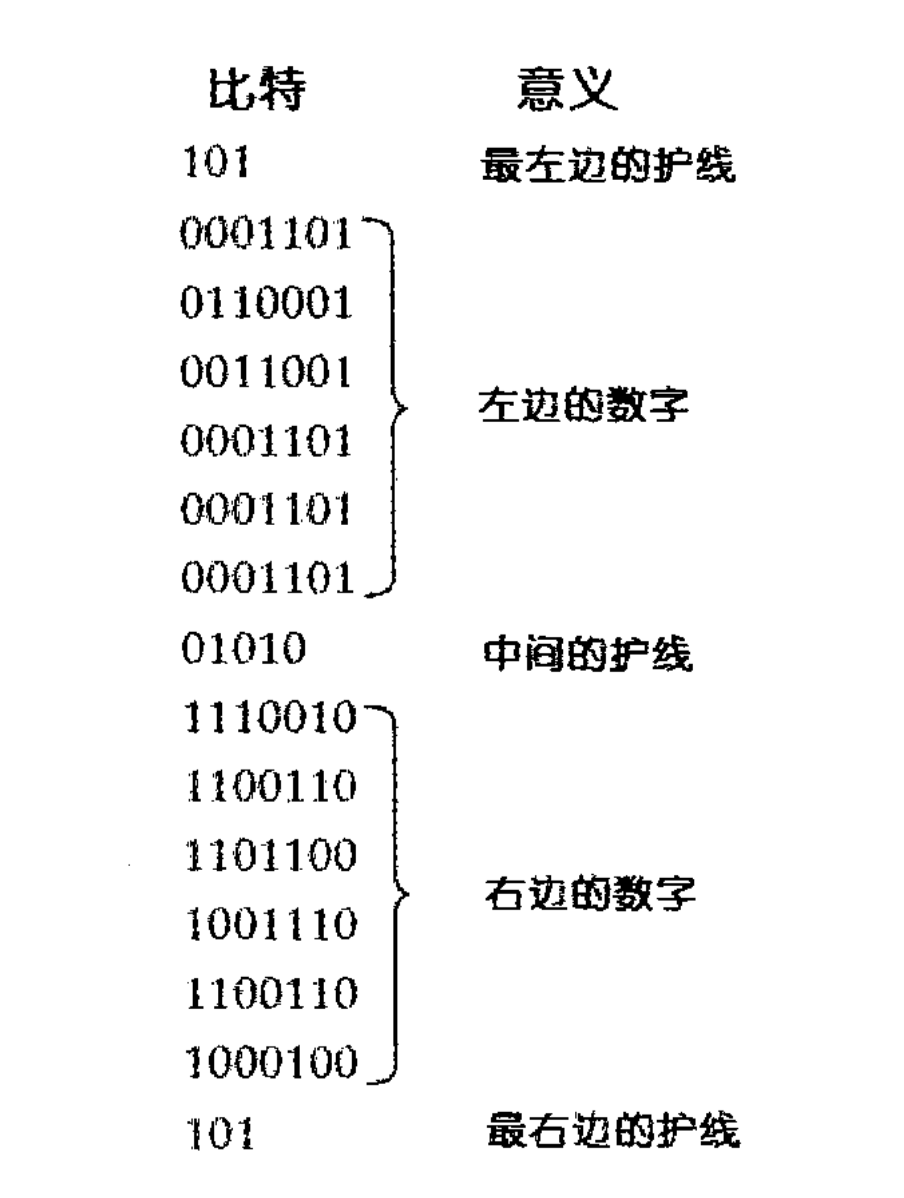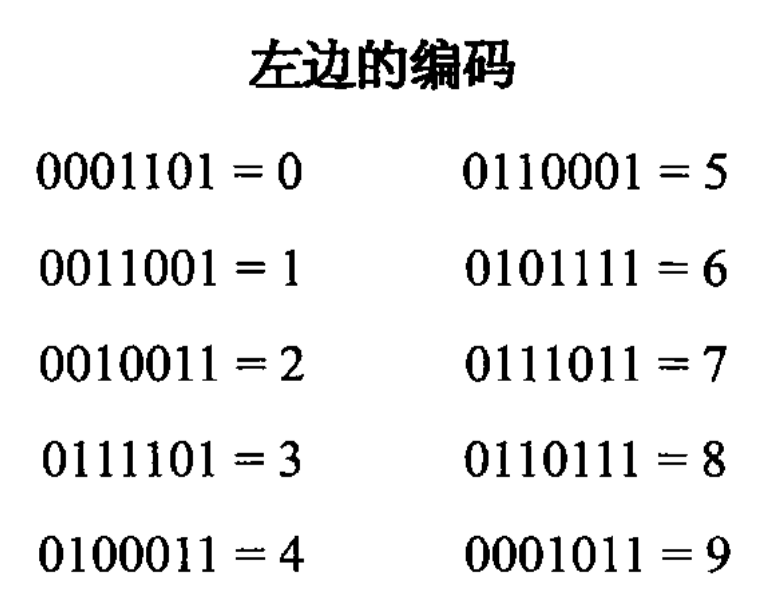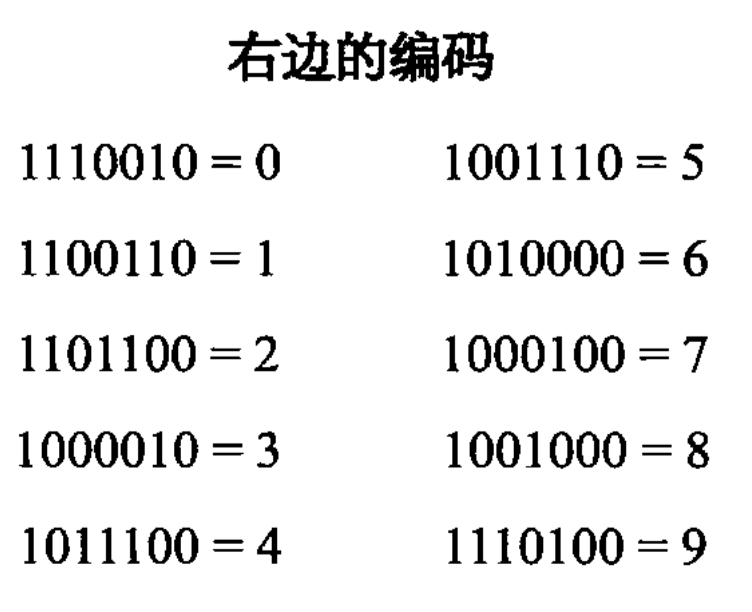A B C D E F G H I J K A \quad BCDEF \quad GHIJK

3 × ( A + C + E + G + I + K ) + ( B + D + F + H + J ) 3 \times (A + C + E + G + I + K) + (B + D + F + H + J)

3 × ( 0 + 1 + 0 + 0 + 2 + 1 ) + ( 5 + 0 + 0 + 1 + 5 ) = 3 × 4 + 11 = 23 3 \times (0 + 1 + 0 + 0 + 2 + 1) + (5 + 0 + 0 + 1 + 5) = 3 \times 4 + 11 = 23

30 − 23 = 7 30 - 23 = 7

UPC可以从两个方向读，这一点是非常方便的。如果扫描装置解码的第一个数是符合偶校验（即7位编码中有偶数个1）的，扫描仪就会知道，它正从右向左扫描UPC码。计算机就会使用如下表来解码：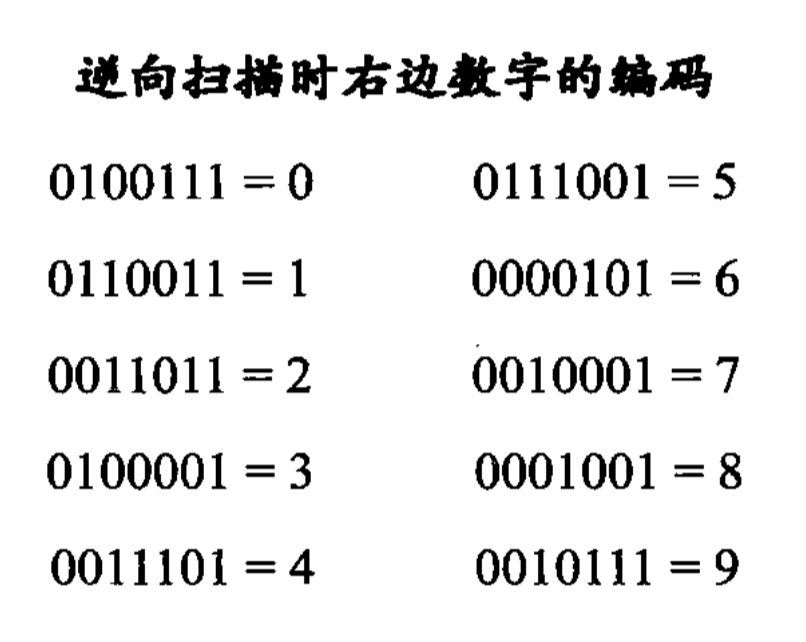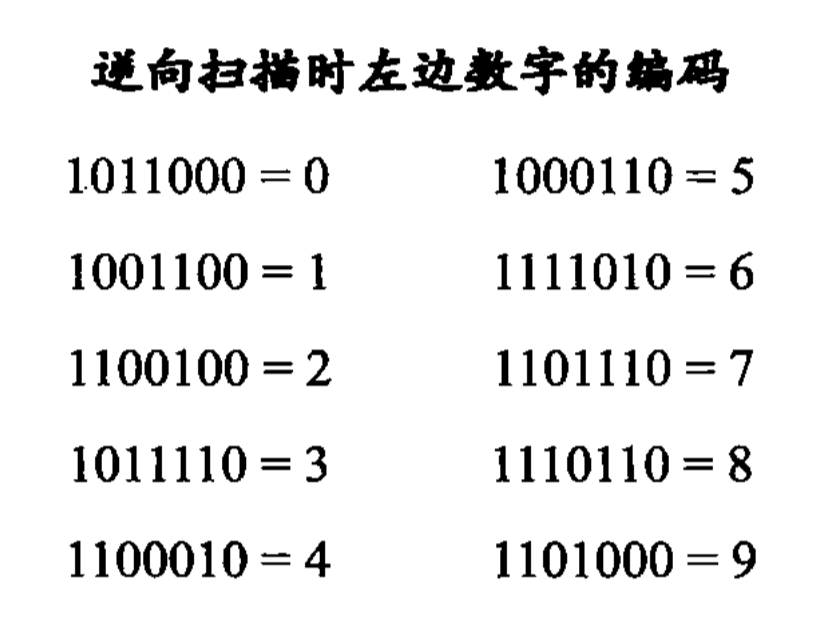## 声明

02-21
07-1408-02410
06-29353
10-301万+
01-144629